﻿ Optimal Decision Number of Times for Search by Approach and Departure with RSSIAmerican Journal of Operational Research

p-ISSN: 2324-6537    e-ISSN: 2324-6545

2020;  10(1): 1-7

doi:10.5923/j.ajor.20201001.01### Optimal Decision Number of Times for Search by Approach and Departure with RSSI

Kazuyuki Ishii , Naoshi Sato , Toshihiro Matsui

Institute of Information Security 2-14-1 Tsuruya-cho, Kanagawa-ku, Yokohama-city, Kanagawa, Japan

Correspondence to: Kazuyuki Ishii , Institute of Information Security 2-14-1 Tsuruya-cho, Kanagawa-ku, Yokohama-city, Kanagawa, Japan.
 Email: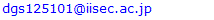Abstract

To study to take the right information from fluctuated data, we take a scenario of searching the signal source by the received signal strength indicator (RSSI). We proposed the moratorium steps for the decision (MSD) to protect the decision until the defined number of times. The evaluation was simulated with fluctuated RSSI and two wireless nodes were simulated. One node broadcasted the signaling and another node received this signaling and measured RSSI. During the moving, RSSI was measured per regular distance. Due to observing the RSSI's increasing multiple times in a row or de-creasing multiple times in a row, the decision can make whether to approach to the signal source or not. In the simulation, the proposed method and the existing proposal were implemented, and the delay performance was compared. As a result, the proposed method was obviously a higher performance of about a maximum of 30 times. Therefore, it was evaluated that it could be good to protect the decision until the defined number of times taking the right information from fluctuated data.

Keywords: Search, Approach and Departure Decision, RSSI, GPS-free, Efficiency, Optimal decision number of times

Cite this paper: Kazuyuki Ishii , Naoshi Sato , Toshihiro Matsui , Optimal Decision Number of Times for Search by Approach and Departure with RSSI, American Journal of Operational Research, Vol. 10 No. 1, 2020, pp. 1-7. doi: 10.5923/j.ajor.20201001.01.

### 1. Introduction

#### 1.1. Background and Motivation

It is important to take the right information from fluctuated data. By defining the rule, the right information has been taken from mass data. This rule wants to be defined objectively. We have studied the decision rule from the fluctuated data and the rule is based on the same observation multiple times . Therefore, in this study we study the scenario of searching the signal source by the received signal strength indicator (RSSI) because RSSI is fluctuated based on the environments and RSSI can be used the decision of approach or departure so that RSSI is an ordinal scale that is inversely proportional to the square of communication distance in theory and the magnitude relationship of the RSSI represents distance . In this paper, we focus on the RSSI which can be obtained during wireless communication . And the scenario is based on ad-hoc communication, which can enable communication between wireless devices. In this paper, the signal source node is called the target node (TN), which broadcast periodically a beacon per certain times. The node to search TN by RSSI of the beacon from TN is the mobile node (MN).

#### 1.2. Problem Statement

We consider the following problems:
1. It is difﬁcult to determine approach or departure, i.e., moving toward or away from TN, respectively, by comparing between RSSI because they can change signiﬁcantly in the same environment due to seasonal and weather changes .
2. It is difﬁcult to estimate the direction of arrival (DoA ) because wireless devices employ non-directional antenna.
For these reasons, it is difﬁcult for MN to search TN when the distance between MN and TN and the direction of TN’s signal are unknown.

#### 1.3. Objective

When moving to a TN under fluctuated RSSI depending on measurement points, a protection feature is implemented to determine approach/departure (approach/departure decision). We propose the moratorium steps for the decision (MSD) as a protection feature to reduce movement distance. To study an MN moving to a TN based on RSSI, we assume that MN and TN can communicate directly. In this study, we evaluate that MN search TN using the MSD through computer simulation.
The reminder of this paper is organized as follows. Section 2 discusses related work. Section 3 describes the proposed method, including an overview, details, and a description of our computer simulation model. Approach/departure decision and the MSD are also discussed in the Section 3. Section 4 presents various simulation results, including a comparison between the proposed method and unused it. The conclusions are given in Section 5.

### 2. Related Work

(a) Estimation of distance based on RSSI.
Many studies of positioning techniques using wireless devices have been conducted. In these studies, distance is estimated by trilateration    , which estimates the distance of an objective node while measuring the distance from more than three anchor nodes. Trilateration is prone to error in distance estimation due to error in the measured RSSI. The trilateration method requires more than three anchor nodes, whereas the proposed method uses only two nodes to search TN.
(b) Estimation of AOA with RSSI .
This method is the combination of two method. The angle measurement and the distance measurement are equally important in determining the position of the target node. It converts polar coordinates into Cartesian coordinates to locate the target node T with respect to the anchor node A as below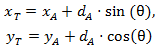where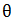is the AOA of signal which is transmitted from the target node T and arrives at node A. The advantage of this method is that we can locate nodes with a minimum of one anchor node. However, AOA reply on special hardware that is expensive and energy consuming. Figure 1 shows the graphical representation of AOA with RSSI.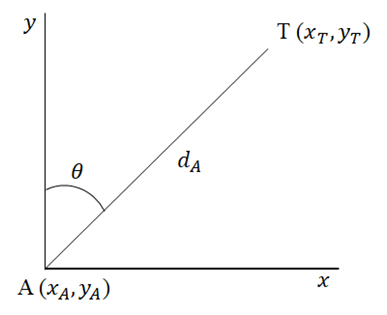Figure 1. Graphical Representation of AOA with RSSI
We review studies about using mobile robots for search and rescue. Such mobile robots search TN using sensor nodes . The mobile robot moves randomly from q0 and stops at one of q1, q2, q3 and q4 that is far away to TN than q1. The movement tracking are deﬁned by the initial distance |q0S| to TN, and the moving distance is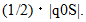The mobile robot moves to this node by heuristic movement following RSSI-based distance estimation, and which is repeated. Until q4 it cannot search S, it implies no progress and it stays at the initial position (q0). Then new iteration is run to search S. Figure 2 shows the sample of heuristics movement with distance noise.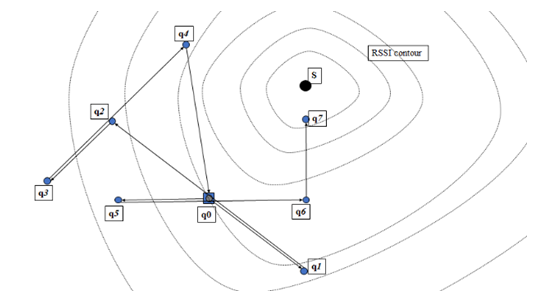Figure 2. Heuristics movement with distance noise in DREAMS
As described above, the related work is described from three perspectives. These studies use trilateration for distance estimation, directional antennas to determine radio direction, and heuristic movement according to RSSI-based distance estimation.

### 3. Proposal Method

#### 3.1. Overview

Since RSSI is fluctuated based on the environment, the calibration of RSSI propagation model  or ﬁltering to remove unwanted components (noise)  and the communication distance is required. This study employs search method that uses approach/departure decision based on fluctuated RSSI from wireless devices with non-directional antennas. The proposed method does not use calibration or ﬁltering to measure RSSI or estimate communication distance. It is difﬁcult to determine approach/departure depending using RSSI strength. The MSD as a protection feature is implemented for approach/departure decision. An overview is shown in Figure 3 assumed on the free-space propagation model without noise to explain easily. When the protection feature is used and the decreasing is determined multiple times in a row, the moving direction changes, that is, approach/departure are determined by decreasing and increasing RSSI, respectively, multiple times in a row, while MN moves in a straight line from a to c. Then, the moving direction is changed at b’ when determining departure using approach/departure decision. With this method, incorrect moving direction is reduced because incorrect approach/departure decision can be prevented. It is that “multiple times in a row” is MSD of a protection feature and MSD is an integer value. We evaluate that MN search TN using the MSD according to approach/departure decision through computer simulation. The notations used in this paper are described in Table 1.
 Table 1. Notations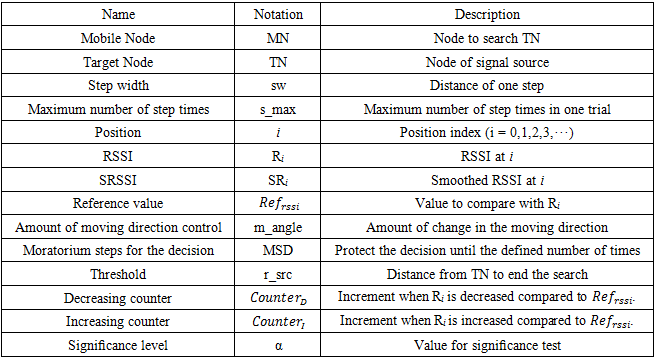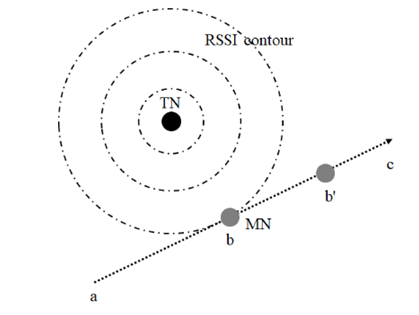Figure 3. Overview of approach/departure decision

#### 3.2. Assumption

MN assumes a general wireless node that implements IEEE 802.11b and supports ad-hoc communication. It is assumed that a non-directional antenna is employed by MN. In addition, MN has the following capabilities:
- Moving distance measurement
- Moving direction control
TN has the same functions and sends a beacon signal to MN periodically.

#### 3.3. Details

Here, we describe TN based on RSSI using approach/departure decision. The search procedure for MN is described below.
(1) MN measures RSSI (Ri) at i from TN.
(2) When starting the search, Ri becomes the reference value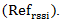(3) MSD is set an integer value (k).
(4) Check whether MN is within r_src. If MN is within r_src, the simulation ends.
(5) Compare Ri to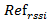.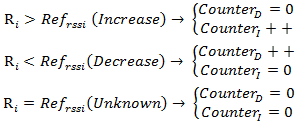(6) Compare each counter with MSD times.
When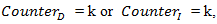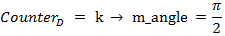or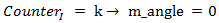then
Go to (7)
else if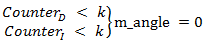then
Go to (8)
(7)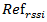is updated and both counters are reset as follows.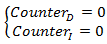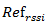is selected as the minimum Ri between the current location (t + N) and the location (t) which is starting the count of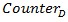or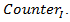In order to prevent from occurring approach determination frequently, the minimum RSSI is selected.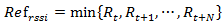(8) Move sw as a single step. One direction is selected when the search process begins.
(9) Repeat (1)
##### 3.3.1. MSD in Approach/Departure Decision
The purpose of approach/departure decision is to determine whether MN moves toward or away from TN according to the measured RSSI at each step. MN compares Ri and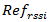to determine the magnitude relation.
MN moves a single step and the probability of decision is 1/2 because the comparison of RSSI is the increased or decreased when comparing Ri to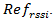We assume that MN determines that the comparison result is increasing or decreasing multiple times in a row. The formula to calculate the multiple times in a row is (0.5)N because increasing or decreasing can occur the propagability of 0.5. Where N is the number of times and the signiﬁcance level (α) is used, which is the probability of rejecting the null hypothesis. Thus, k is the minimum integer value.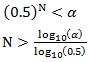(1)
Note that N becomes 5 because α is set to 0.05.
Due to fluctuated RSSI caused by environmental conditions, we assume that the variance of RSSI is used for the MSD. The variance at i is obtained from Ri and the smoothed RSSI (SRi) at i . This is expressed as Di,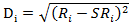(2)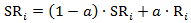(3)
where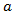is the smoothing constant and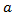is set to 0.125.
Here, we consider N which is calculated statistically and the variance Di. One is a ﬁxed value, and the other is a variable value because it is based on the variance of RSSI which is obtained while MN moves. Thus, the MSD is an integer value selected as the maximum value of N or Di. Note that the MSD at i is deﬁned as below.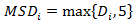(4)
We determine the approach to TN when the comparison Ri with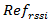is increasing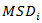times in a row, and we determine departure from TN when he comparison Ri with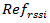is decreasing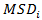times in a row. It is expected that N prevent incorrect determination from the varied Ri, i.e., the measured RSSI.
##### 3.3.2. Moving Direction Control
The moving direction control controls the moving direction according to the approach/departure decision. To increase the possibility of encountering TN, the movement trajectory of MN is a spiral box search, i.e., m_angle is 0 or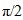in a counterclockwise direction.

### 4. Evaluation

In this section, we evaluate the performance of the proposed method through computer simulation. The proposed method is a generic algorithm that is designed for realistic settings without prior knowledge of sensory filed and with RSSI measurement noise. Only a few mobility scheduling algorithms were presented in the literature. However, none of them is comparable to the proposed method (see Section 2 for a related work). In this case, we decide to use the RSSI-based mobility algorithm for comparison which is DREAMS . The reason is that this algorithm is a heuristic movement as having four attempts of one iteration to compare the RSSI-based distances and it is similar to the proposed method that compares the RSSI within several times of one iteration. Our study focuses on:
Delay performance: It is measured by moving distance divided by distance between the starting point and TN. The former is the total distance that MN moves during the search, the latter is the direct distance until TN from the starting point of search. It is a metric in order to measure how much MN takes a detour toward TN. The metric is detour rate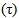described as below.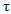has a numeric value that is greater than 1.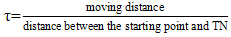(5)

#### 4.1. Plane of Search

The plane of search is a two-dimensional lattice in the x−y plane. The distance between the lattices is 0.5 [m]. MN and TN are positioned on this x−y plane, and it is assumed that there are no obstacles between these nodes.
##### 4.1.1. MN
MN moves at a constant speed to adjacent lattice points on the left, right, top or bottom of the x−y plane. At each lattice point MN determines Ri from TN.
##### 4.1.2. TN
TN is located on at the origin (0,0) at x−y plane and does not move.
TN transmits signals such as a beacon signal, and MN measures Ri. The radio propagation model uses a generic model, i.e., the variations in RSSI follow a log-normal distribution  .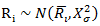(6)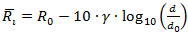(7)
Here, Ri [dBm] is RSSI measured at i and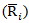[dBm] is the average RSSI at i.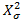[dB] is the variance, i.e., the varied measurements of RSSI.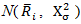indicates a log-normal distribution of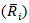and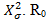[dBm] is RSSI at reference distance d0 [m], and d0 [m] is 1 [m]. Thus, R0 using a free space model  (frequency: 2.4 [GHz], transmitting power: 10 [mW] , antenna gain: 1) as -30.05 [dBm]. d [m] is communication distance. γ is the path loss exponent and it is covered as a fixed value. It is assumed that d and γ are used as unknown parameters. The simulations cover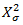of 32 to 122 [dB] because these values are reported in the literature . The parameters are listed in Table 2 in the both simulations.
 Table 2. RSSI parameters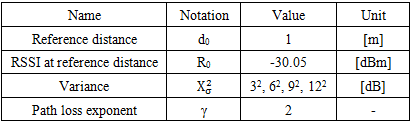#### 4.2. Simulation Setup

We implemented the proposed method and DREAMS  in a custom simulator by Visual C++ 2017. In the both simulations, an MN and TN were positioned on the search space. MN started from the following start points given as an x, y coordinate respectively: {x,y}={100,100}, {90,90}, {80,80}, {70,70}, {60,60}, {50,50}, {40,40}, {30,30}, {20,20} (Unit: [m]). This is a range that MN can receive the signal from TN in roughly maximum -100 [dBm]. TN is allocated at the origin (0,0) at x−y plane and TN is ﬁxed. Table 3 shows the simulation parameters which is used in the both simulations. However, the amount of moving direction control (m_angle) and signiﬁcance level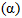were used in only the proposed method. We conducted 100 simulation runs at each starting point. Note that the simulation of DREAMS calculated the distance by Equation (7) with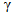in Table 2 and it limited the Maximum moving distance in one time was set to 100 [m] because of avoiding the unrealistic distance calculating by RSSI.
 Table 3. Simulation parameters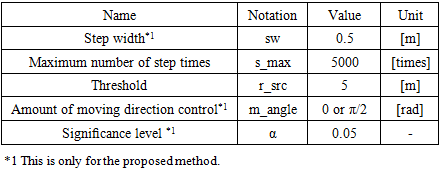#### 4.3. Simulation Result

We evaluate the proposed method and DREAMS  in single-hop scenario. Table 4 shows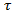in the both simulation results. From the results, the proposed method shows the better result of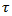than DREAMS. In the proposed method, the results show a shorter distance of about 3 to 40 times depending on X2σ. This can be explained by the fact that the RSSI-based distance causes the wrong distance in DREAMS.
 Table 4.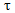of Proposed method and DREAMS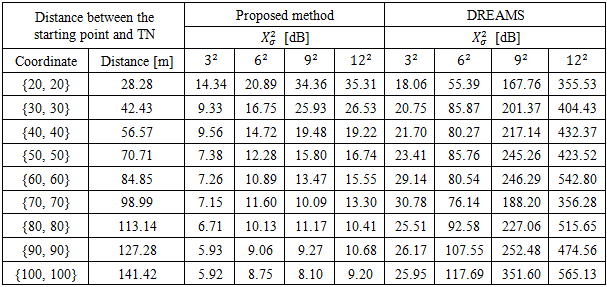In the next, RSSI is a sampling (10 times) at the same position and averaged in DREAMS. This result shows in Table 5. Though DREAMS in Table 5 is the better than one in Table 4, the proposed method presents the better result than DREAMS with the averaged RSSI. However, in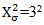[dB] and {20, 20}, DREAMS is better than the proposed method about 1.4 times. This is only one case in which DREAMS is better. Other cases are the shorter distance of about 1.7 to 30 times. Figure 4 shows one example of movement locus (Position i on x-axis, MSDi on y-axis) in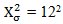[dB]. Though MN had several wrong decisions, the direction was recovered. In Figure 4, MN started the search at Start Point of MN of {x,y}={100,100} and had a wrong decision at (B) and changed the direction and moved until (C). At (C), (D) and (E) MN detected departure from TN due to that the comparison was decreasing MSDi time in a row. Then, the direction was recovered. Later, there were the same procedures which contains a wrong decision at (G). Finally, MN searched TN because of arriving within r_src from TN. Since MSDi became the bigger value because of the high variance environment, MN consumed the movement until the decision of approach or departure.
 Table 5.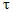of Proposed method and DREAMS with averaged RSSI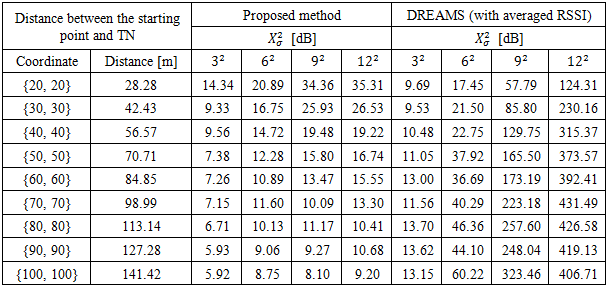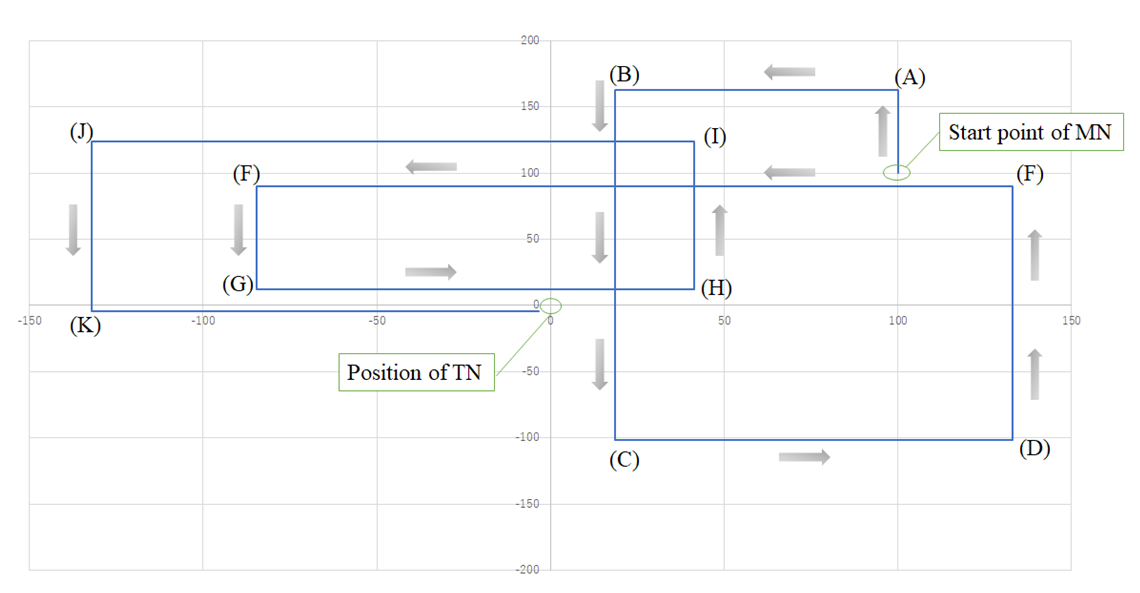Figure 4. Example of movement locus
This is worth nothing that delay performance greatly depends on RSSI-based distance accuracy. And this is a key point that the proposed method is using the moratorium steps for the decision and it is independent of RSSI-based distance accuracy.

### 5. Conclusions

The search based on the moratorium steps for the decision (MSD) is a cost-effective search in delay tolerant scenario. In this paper, we used the search of signal source by fluctuated RSSI scenario to evaluate the effective search using the effective steps for a decision. We studied how to avoid the mis decision under fluctuated RSSI. We proposed the moratorium steps for the decision, protecting the decision until the multiple times. The evaluation was performed under fluctuated RSSI to search the signal source in the simulation. Our simulation results imply that the proposed method using the moratorium steps for the decision has better the delay performance than the existing proposal DREAMS  at reasonable delay cost. The different results from the both were whether to consider the variance of RSSI. It indicated that the proposed method could make the better decision under fluctuated RSSI.
In this paper, it was handled a simple way such as a single-hop scenario, no movement of target or no obstacle environments. When MN cannot receive instructions from TN, it starts a random walk to discover the area which can be got instructions from TN. Once it is found, MN runs the proposed method from there. By this means, it is a limitation of a single-hop scenario and it implies that a multi-hop scenario can be solved. It is a challenging task to enable moving TN which means that variance of RSSI is so high and it is required to take the right information from fluctuated data. And a multi-hop scenario means considering the organized search including the task of moving TN for further investigation. Finally, it is expected that this study helps to take the right information from fluctuated data.

### References

  K.Ishii, N. Sato, “Approaching a Target using a Protection Feature based on Received Signal Strength Indicator,” In Proceedings of 5th the International Conference on Operations Research and Enterprise Systems (ICORES 2016), pp. 170-177, 2016.  Rappaport TS, et al, “Wireless communications: principles and practice,” Vol. 2, PTR New Jersey, 1998.  G. Mao, BD. O. Anderson, B. Fidan, “Path loss exponent estimation for wireless sensor network localization”, Computer Networks, Vol. 51, Issue 10, pp. 2467-2483, 2007.  P.N. Pathirana, N. Bulusu, A.V. Savkin S. Jha, “Node localization using mobile robots in delay-tolerant sensor networks,” IEEE Transactions on Mobile Computing, Vol. 4, Issue 3, pp. 285-296, 2005.  H.T. Friis, “A Note on a Simple Transmission Formula,” In Proceedings of the IRE, Vol. 34, Issue 5, 1946.  S. Capkun, M. Hamdi, J.-P. Hubaux, “GPS-free positioning in mobile ad-hoc networks,” In Proceedings of the 34th Annual Hawaii International Conference on System Sciences, 2001.  D. Niculescu , B. Nath, ” Ad hoc positioning system (APS) using AOA,” In INFOCOM 2003, Vol. 3, pp. 1734–1743, 2003.  D. Niculescu , B. Nath, ” Ad hoc positioning system (APS),” In GLOBECOM'01, Vol. 5, pp. 2926-2931, 2001.  Xu Li, Nathalie Mitton, Isabelle Simplot-Ryl, David Simplot-Ryl, “Dynamic Beacon Mobility Scheduling for Sensor Localization,” IEEE Transactions on Parallel and Distributed Systems, Vol. 23, Issue 8, 2012.  K.Fall, K. Varadhan, ” The ns manual (formerly ns Notes and Documentation),” The VINT Project, https://www.isi.edu/nsnam/ns/doc/index.html, 2011.  CK Toh, “Ad hoc mobile wireless networks: protocols and systems,” Pearson Education, 2001.  B. Priyantha, A. Miu, H. Balakrishnan, S. Teller, “The Cricket Compass for Context-Aware Mobile Applications,” In Proceedings of 7th ACM MOBICOM, pp. 1-14, 2001.  B. R. Jackson, S. Rajan, B. J. Liao, and S. Wang, “ Direction of arrival estimation using directive antennas in uniform circular arrays,” IEEE Transactions on Antennas and Propagation, Vol. 63, Issue 2, pp. 736–747, 2015.  D.Porcino, W. Hirt, “Ultra-wideband radio technology: Potential and challenges ahead,” IEEE Communications Magazine, Vol. 41, Issue 7, pp. 66-74, 2003.  N.M. Ngyenet al., "Performance Evaluation of Non-GPS Based Localization Techniques under Shadowing Effects," Sensors, vol. 19, pp. 2633-1-2633-21, 2019.